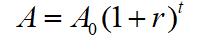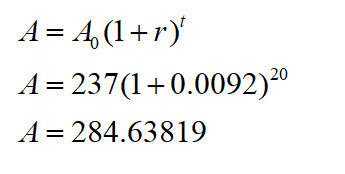# A​ country's population​ (in millions) and the growth rate​ (%) in 2015 are given below. Use this information and an exponential model to estimate the​ country's population in the specified year.population=237 ​milliongrowth rate=0.92%year, 2035The​ country's population in 2035 will be ____million?(Round to the nearest hundredth as needed.)

Question
1 views

A​ country's population​ (in millions) and the growth rate​ (%) in 2015 are given below. Use this information and an exponential model to estimate the​ country's population in the specified year.

population=237 ​million

growth rate=0.92%

year, 2035

The​ country's population in 2035 will be ____million?

(Round to the nearest hundredth as needed.)

check_circle

Step 1

Exponential growth formula isStep 2

Let ‘t’ be the number of year since 2015. In 2015, the value of t=0,  and In 2035 the value of t=20

Initial population is 237  and growth rate r= 0.92%= 0.0092, t=20

Step 3

Plug in the values in the formula and find out A...

### Want to see the full answer?

See Solution

#### Want to see this answer and more?

Solutions are written by subject experts who are available 24/7. Questions are typically answered within 1 hour.*

See Solution
*Response times may vary by subject and question.
Tagged in

### Algebra